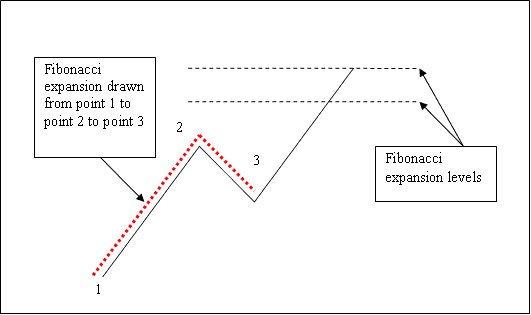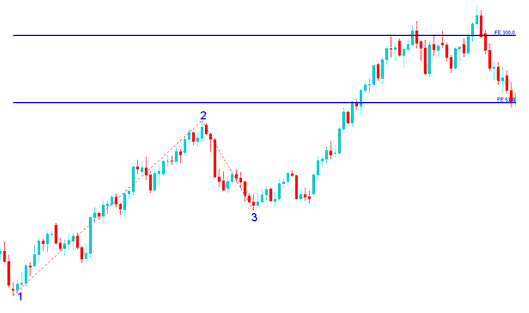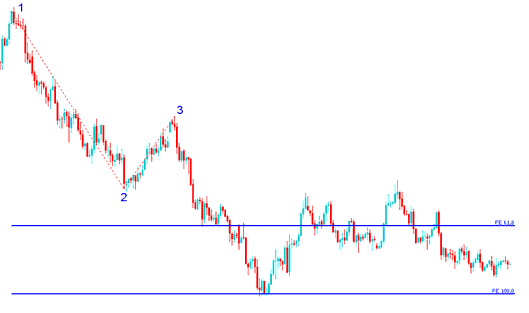# How to Draw Indices Trading Fibonacci Extension Indicator Tool on Stock Indexes Charts

## How Do I Use Indices Trading Fibonacci Extension Levels on Indices Chart?

We use Indices Trading Fibonacci Extensionlevels to estimate where the indices trend movement will reach. There are 2 important Indices Trading Fibonacci Extension levels; 61.8% and 100% Indices Trading Fibonacci Extension Levels, these are used for taking profit.

To draw Indices Trading Fibonacci Extension levels on the stock indices chart we wait until the stock indexes price retracement is complete and the stock indexes price starts to move in the original direction of the Indices trend. Where the stock indexes price retracement reaches is used as chart point 3. Indices Trading Fibonacci Extension Indicator is drawn using three chart points as shown on the stock indexes trading example explained and illustrated below of how to draw the Indices Trading Fibonacci Extension Indicator Tool.

The Indices Trading Fibonacci Extension example explained and illustrated below shows the 3 Chart Points where the Indices Trading Fibonacci Extension indicator is drawn, marked as Chart point 1, Chart point 2 and Chart point 3.

Chart point 1 is where the indices trend started, Chart point 2 is where the indices trend pulled back and retraced and Chart point 3 is where the stock indices retracement reached as shown on the Indices Trading Fibonacci Extension Indicator Tool example explained and illustrated below.How To Trade With Indices Trading Fibonacci Extension? - How to Draw Indices Trading Fibonacci Extension Levels on Indices Charts

Please note where these Indices Trading Fibonacci Extension levels are drawn - Indices Trading Fibonacci Extension levels are drawn above the Fibonacci indicator, these are the chart points where a indices trader will set the take profit orders using these Indices Trading Fibonacci Extension Levels - 61.8% and 100.0% Indices Trading Fibonacci Extension Levels.

## Drawing Indices Trading Fibonacci Extension Levels on an Upward Indices Trend

We use Indices Trading Fibonacci Extensionlevels to estimate where the indices trend movement will reach. There are 2 important Indices Trading Fibonacci Extension levels; 61.8% Indices Trading Fibonacci Extension Level and 100% Indices Trading Fibonacci Extension Level, these Indices Trading Fibonacci Extension Levels are used for taking profit.

On the Indices Trading Fibonacci Extension indicator example explained and illustrated below you can see that the Indices Trading Fibonacci Extension indicator tool is drawn along the direction of the indices trend, since the indices trend is upwards - the Indices Trading Fibonacci Extension indicator is drawn upwards.

These Indices Trading Fibonacci Extension levels are displayed as horizontal lines above the Indices Trading Fibonacci Extension indicator, showing profit taking areas. In the stock indexes trading example explained and illustrated below if you had used of 100.0% Indices Trading Fibonacci Extension level you would have made nice profit from the trade setup.Drawing Indices Trading Fibonacci Extension Indicator on an Upward Indices Trend - Indices Trading Fibonacci Extension Trading Tool

From the above Indices Trading Fibonacci Extension indicator example, the upward indices trend continued and both 61.8% and 100.0% Indices Trading Fibonacci Extension levels were all hit after which stock indexes price retraced again after getting to the 100.0 % Indices Trading Fibonacci Extension level.

## Drawing Indices Trading Fibonacci Extension Levels on a Downward Indices Trend

Since we use the Indices Trading Fibonacci Extension indicator tool to estimate take profit levels, how do we draw Indices Trading Fibonacci Extension in a downward Indices trend?

We draw the Indices Trading Fibonacci Extension indicator tool from chart point 1 to chart point 2 to chart point 3 as shown on the stock indexes trading example explained and illustrated below. Remember we always draw this Indices Trading Fibonacci Extension tool in the direction of the indices trend. In the Indices Trading Fibonacci Extension example explained and illustrated below, can you figure what direction we have drawn it? That is right - downward direction.

Try and spot the difference between how we have drawn Indices Trading Fibonacci Extension indicator above and how Indices Trading Fibonacci Extension indicator is drawn below. This time you would also have used Indices Trading Fibonacci Extension level 100.0%, just where the stock indexes price reached as shown on the stock indexes trading example explained and illustrated below. That would have been a good take profit area.Drawing Indices Trading Fibonacci Extension Indicator on a Downward Indices Trend - Indices Trading Fibonacci Extension Trading Tool

From the Indices Trading Fibonacci Extension indicator example above, after drawing this Indices Trading Fibonacci Extension tool there are two levels that are used to show the profit taking areas, these two Indices Trading Fibonacci Extension levels are drawn as horizontal lines across the stock indexes price chart marked as 61.8% Indices Trading Fibonacci Extension Level and 100% Indices Trading Fibonacci Extension Level.